# How to AutoSum Multiple Rows/Columns/Worksheets in Excel?

When we are performing a sum for a single column or row in Excel, we can directly use the formula to do it, but if we are doing the same process for multiple rows or columns at once, we cannot do it using the formulas. We can complete the process by using the auto function in Excel. This tutorial will help you understand how we can autosum multiple rows, columns, or worksheets in Excel.

## AutoSum Multiple Rows in Excel

Here we will use the autosum function under formulas. Let us see a straightforward process to see how we can autosum multiple rows at once in Excel.

Step 1

Consider the following image of an Excel sheet containing numbers in multiple rows.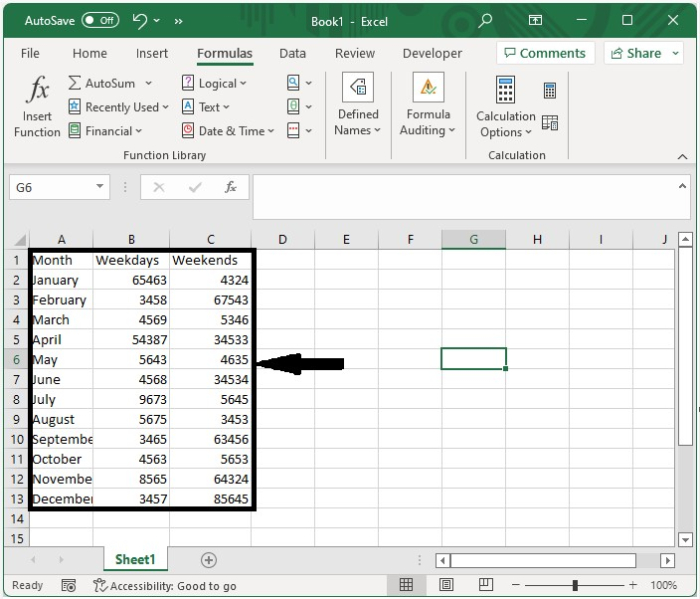Now to perform the autosum. Select the data, click on formulas, and then click on autosum, as shown in the below image.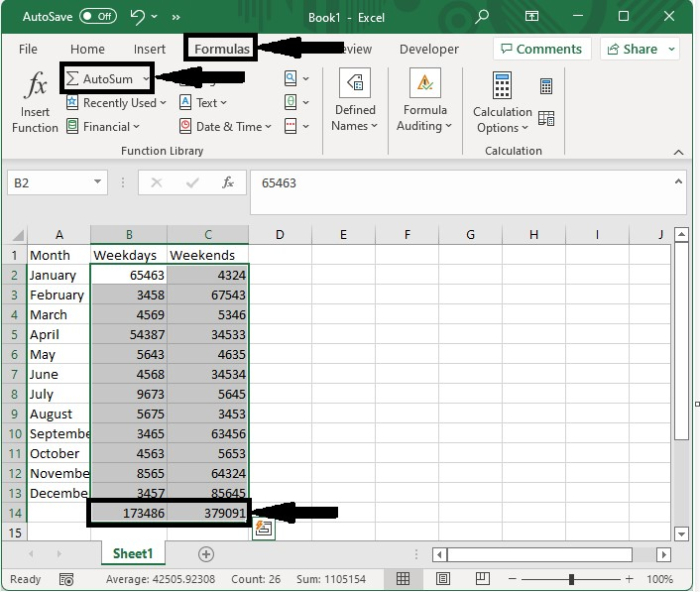## AutoSum Multiple Columns in Excel

Here we will first select the range of cells and use the autosum function. Let us see an uncomplicated process to see how we can autosum multiple columns at once in Excel.

Step 1

Let us consider the same data that we considered in the above example, select the data along with an extra column, and click on auto-sum to complete our process.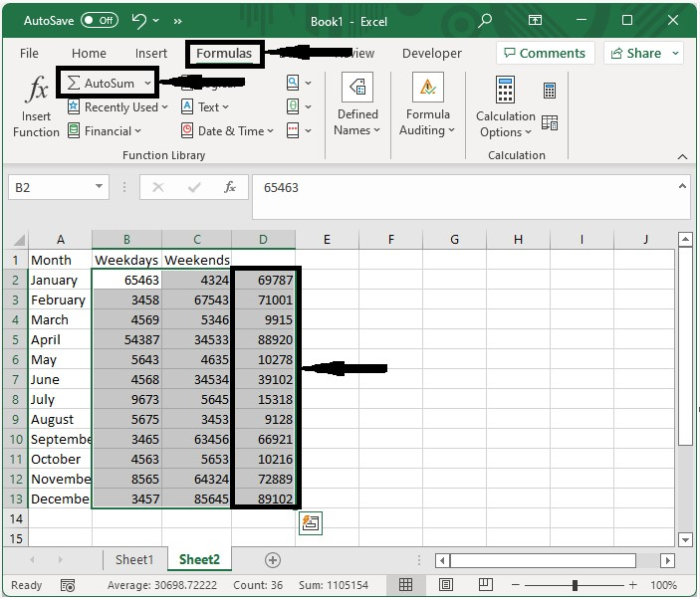Step 2

We can also perform the sum of multiple rows and columns at once; to do that, select the data along with an extra column and row and click on "Autosum" under the formulas.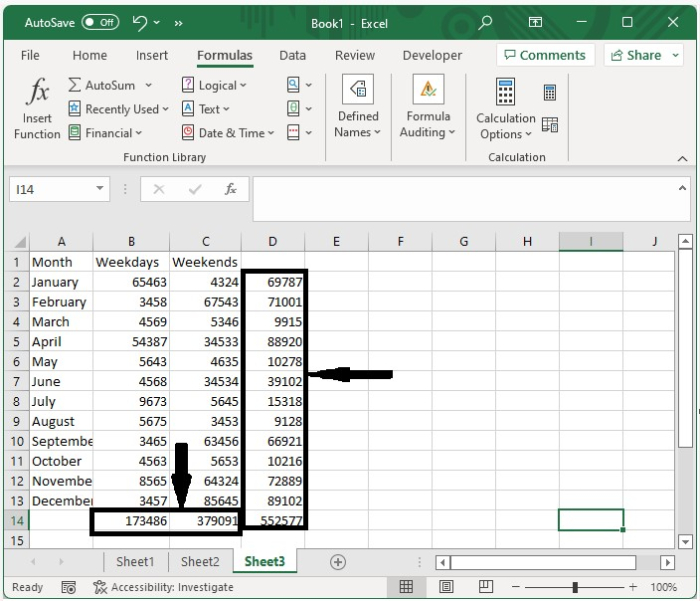## AutoSum Multiple Worksheets in Excel

Here we will use the SUM formula to add data from multiple sheets. Let's go over an easy way to understand how to autosum multiple worksheets in Excel.

Step 1

Let us consider multiple sheets that contain numbers, as shown in the below images.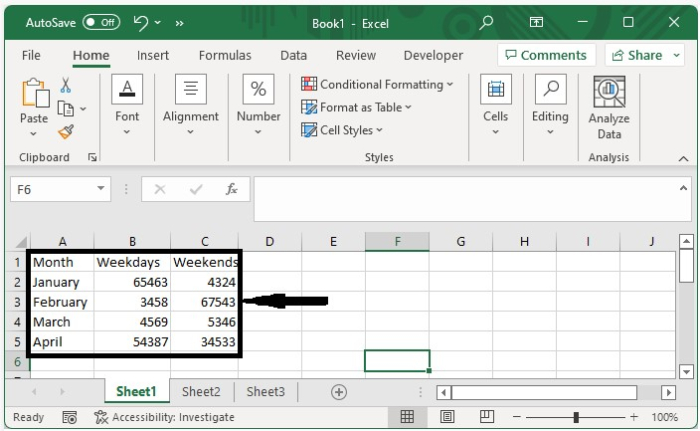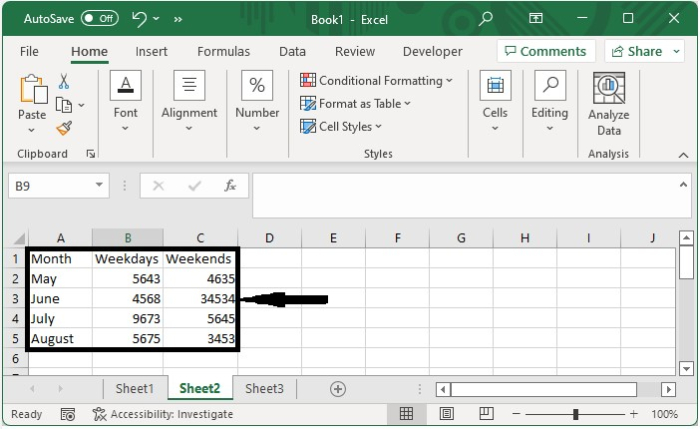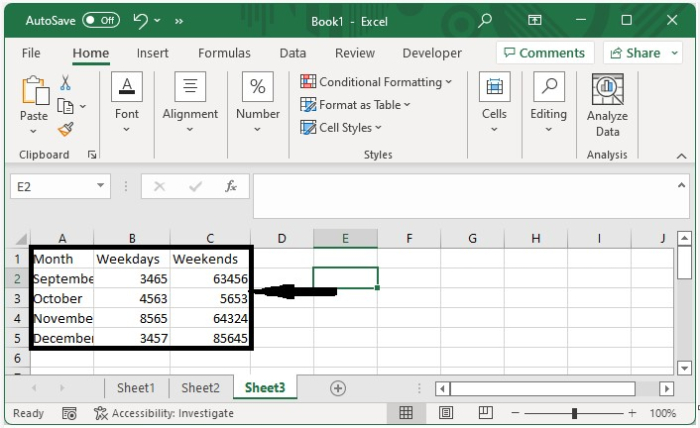We can sum all the values in multiple worksheets using the formula =SUM(Sheet1:Sheet3!B2:C5), then press Enter to get the result shown in the image below.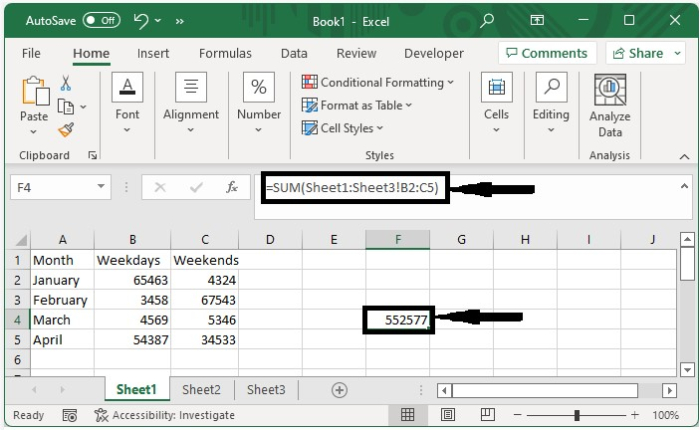## Conclusion

In this tutorial, we used a simple example to demonstrate how we can auto-sum multiple rows, columns, and worksheets in Excel.

Updated on: 12-Jan-2023

178 Views